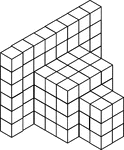### 108 Stacked Congruent Cubes

Illustration of 108 congruent cubes stacked at various heights. A 3-dimensional representation on a…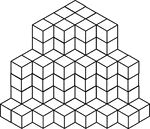### 117 Stacked Congruent Cubes

Illustration of 117 congruent cubes stacked in columns of one, four, and six. A 3-dimensional representation…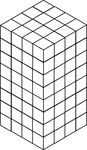### 128 Stacked Congruent Cubes

Illustration of 128 congruent cubes stacked so they form a rectangular solid that measures 4 by 4 by…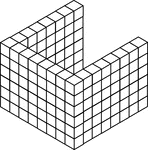### 132 Stacked Congruent Cubes

Illustration of 132 congruent cubes stacked in 22 columns of 6 in the shape of a U. A 3-dimensional…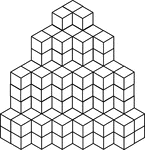### 154 Stacked Congruent Cubes

Illustration of 154 congruent cubes stacked in columns increasing from one to four. A 3-dimensional…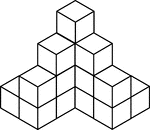### 16 Stacked Congruent Cubes

Illustration of 16 congruent cubes stacked at various heights. A 3-dimensional representation on a 2-dimensional…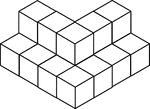### 17 Stacked Congruent Cubes

Illustration of 17 congruent cubes stacked in ones and twos in the shape of a V. A 3-dimensional representation…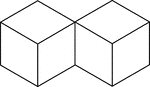### 2 Congruent Cubes

Illustration of two congruent cubes that are tangent along an edge. A 3-dimensional representation on…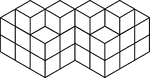### 20 Stacked Congruent Cubes

Illustration of 20 congruent cubes stacked in twos and threes. A 3-dimensional representation on a 2-dimensional…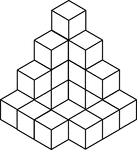### 20 Stacked Congruent Cubes

Illustration of 20 congruent cubes stacked at various heights. A 3-dimensional representation on a 2-dimensional…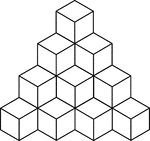### 20 Stacked Congruent Cubes

Illustration of 20 congruent cubes stacked at heights increasing from 1 to 4 cubes. A 3-dimensional…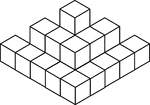### 22 Stacked Congruent Cubes

Illustration of 22 congruent cubes stacked in ones, twos, and threes. A 3-dimensional representation…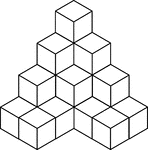### 22 Stacked Congruent Cubes

Illustration of 22 congruent cubes stacked at various heights. A 3-dimensional representation on a 2-dimensional…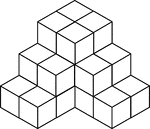### 24 Stacked Congruent Cubes

Illustration of 24 congruent cubes stacked at various heights to resemble steps. A 3-dimensional representation…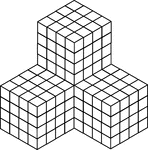### 256 Stacked Congruent Cubes

Illustration of 256 congruent cubes stacked so they form 4 larger cubes that measures 4 by 4 by 4 each.…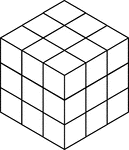### 27 Stacked Congruent Cubes

Illustration of 27 congruent cubes stacked to resemble a larger cube that measures three by three by…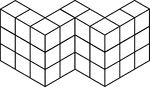### 27 Stacked Congruent Cubes

Illustration of 27 congruent cubes stacked at various heights in the shape of a W. A 3-dimensional representation…### 28 Congruent Cubes Placed in the Shape of a Square

Illustration of 28 congruent cubes placed in the shape of a square. A 3-dimensional representation on…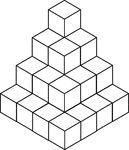### 30 Stacked Congruent Cubes

Illustration of 30 congruent cubes stacked in decreasing heights. A 3-dimensional representation on…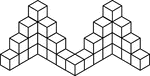### 33 Stacked Congruent Cubes

Illustration of 33 congruent cubes stacked at various heights in a zigzag pattern. A 3-dimensional representation…### 35 Stacked Congruent Cubes

Illustration of 35 congruent cubes stacked in ones and twos in the shape of a W. A 3-dimensional representation…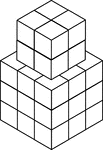### 35 Stacked Congruent Cubes

Illustration of 35 congruent cubes stacked at various heights. A 3-dimensional representation on a 2-dimensional…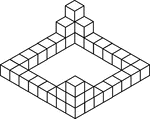### 36 Stacked Congruent Cubes

Illustration of 36 congruent cubes stacked at various heights with outer edges forming a square. A 3-dimensional…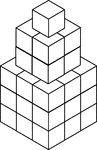### 36 Stacked Congruent Cubes

Illustration of 36 congruent cubes stacked to resemble a 1 by 1 by 1 cube on a 2 by 2 by 2 cube on a…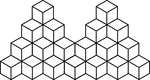### 39 Stacked Congruent Cubes

Illustration of 39 congruent cubes stacked at various heights. A 3-dimensional representation on a 2-dimensional…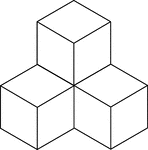### 4 Stacked Congruent Cubes

Illustration of 4 congruent cubes stacked in ones and twos. A 3-dimensional representation on a 2-dimensional…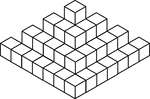### 50 Stacked Congruent Cubes

Illustration of 50 congruent cubes stacked at various heights. A 3-dimensional representation on a 2-dimensional…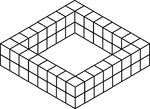### 56 Stacked Congruent Cubes

Illustration of 56 congruent cubes stacked in twos in the shape of a square. A 3-dimensional representation…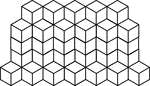### 56 Stacked Congruent Cubes

Illustration of 56 congruent cubes stacked in heights of 1, 4, and 5 cubes that form a zigzag pattern.…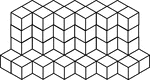### 57 Stacked Congruent Cubes

Illustration of 57 congruent cubes stacked in heights of 1 and 5 cubes that form a zigzag pattern. A…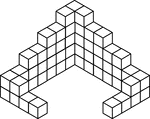### 59 Stacked Congruent Cubes

Illustration of 59 congruent cubes stacked at various heights. A 3-dimensional representation on a 2-dimensional…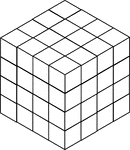### 64 Stacked Congruent Cubes

Illustration of 64 congruent cubes stacked so they form a cube that measures 4 by 4 by 4. A 3-dimensional…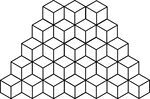### 65 Stacked Congruent Cubes

Illustration of 65 congruent cubes stacked at heights increasing from 1 to 5 cubes. A 3-dimensional…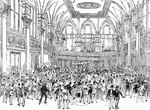### New York Stock Exchange

New York Stock Exchange trading floor.